Google 和 Nvidia 聯手，推出最佳化版的 TensorFlow 1.7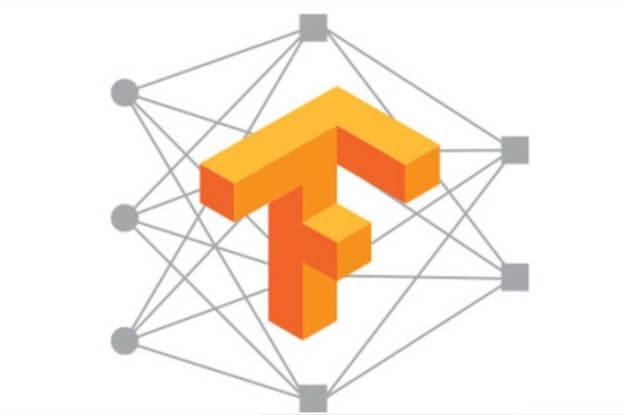ensorRT 是一個可以用於最佳化深度學習模型，以進行推理，並為生產環境的 GPU 建立執行環境的程式庫。它能最佳化 TensorFlow FP16 浮點數和 INT8 整型數，並能自動選擇針對特定平台的核心，以最大化吞吐量，並最大限度的降低 GPU 推理期間的延遲。全新的整合工作流程簡化了 TensorFlow 使用 TensorRT 的步驟，同時使 TensorFlow 達到世界一流的效能水準。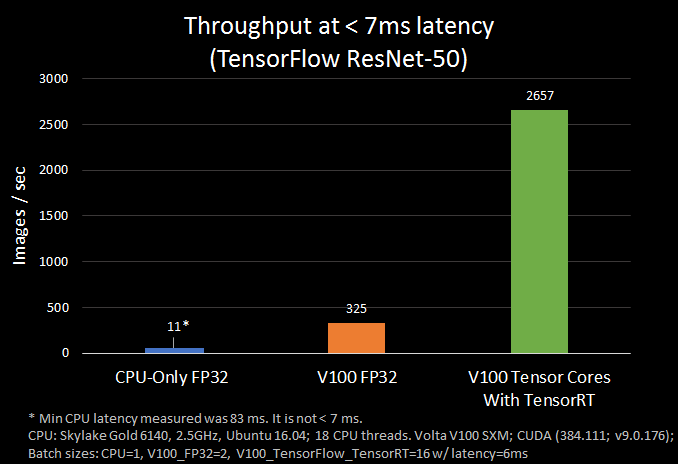最佳化 TensorFlow 的子圖

ensorFlow 1.7 中，TensorRT 可用於最佳化子圖，而 TensorFlow 執行其餘未最佳化的部分。這個方法使開發者既能使用 TensorFlow 的眾多功能快速構建模型，同時也可在執行推理時使用 TensorRT 獲得強大的最佳化能力。如果你試過在之前 TensorFlow 模型使用 TensorRT，你應該知道，要使用某些不受支援的 TensorFlow 層，必須手動導入，這在某些情況下可能會耗費大量時間。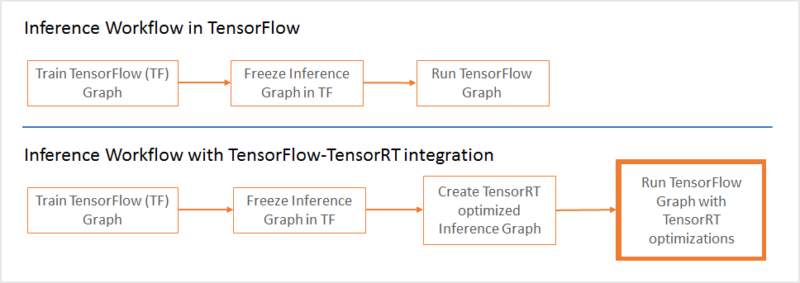# Reserve memory for TensorRT inference engine

gpu_options = tf.GPUOptions(per_process_gpu_memory_fraction = number_between_0_and_1)

…

trt_graph = trt.create_inference_graph(

input_graph_def = frozen_graph_def,

outputs = output_node_name,

max_batch_size=batch_size,

max_workspace_size_bytes=workspace_size,

precision_mode=precision)  # Get optimized graph

per_process_gpu_memory_fraction 這個參數定義了 TensorFlow 允許使用的 GPU 顯存比例，剩餘的顯存將配置給 TensorRT。這個參數應在 TensorFlow-TensorRT 程式第一次啟動的時候設定好。比如 per_process_gpu_fraction=0.67，那麼 67% 的顯存會配置給 TensorFlow，其餘 33% 會配置給 TensorRT 引擎。

Create_inference_graph 函數將凍結的 TensorFlow 圖為匯入，傳回一個經過 TensorRT 節點最佳化的圖。看看這個函數的參數：

• Input_graph_def凍結的 TensorFlow 圖。
• Outputs匯出節點名字的字串清單，比如：[“resnet_v1_50/predictions/Resape_1”]。
• Max_batch_size整數，匯入的 batch size，比如 16。
• Max_workspace_size_bytes整數，能配置給 TensorRT 的最大 GPU 顯存。
• Precision_mode字串，可選的值為「FP32」「FP16」「INT8」。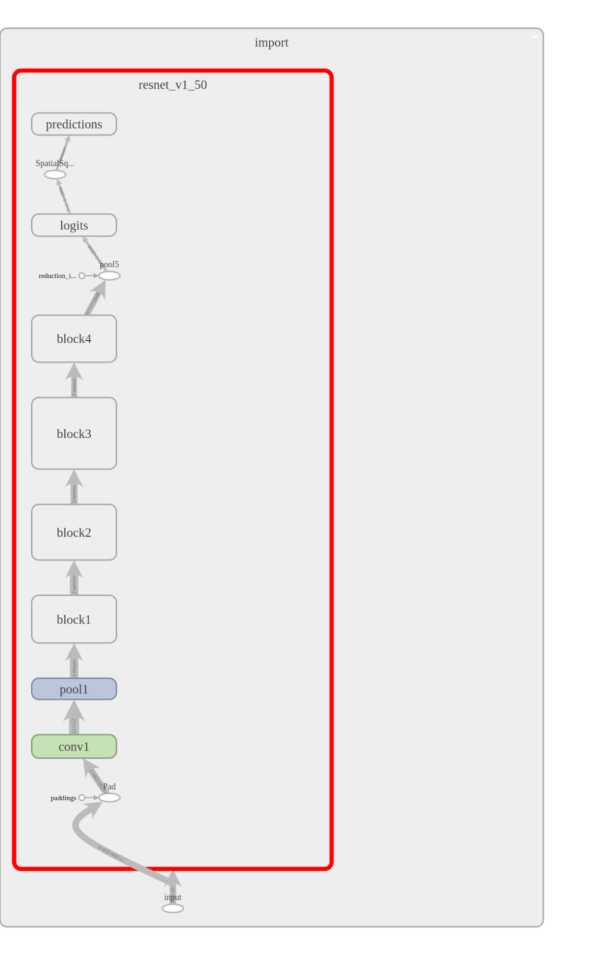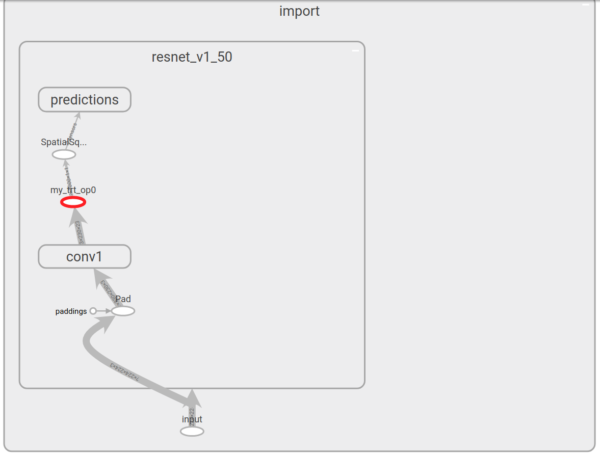經過最佳化的 INT8 推理效能

TensorRT 相容單精準度（FP32）和半精度（FP16）訓練的模型（也可以將它們量化為 INT8），同時盡可能減少由精確度降低導致的準確率降低。INT8 模型能更快計算，同時對頻寬的需求也會降低，但因可用的動態範圍降低，這也對神經網路的權重和觸發表示提出了很大的挑戰。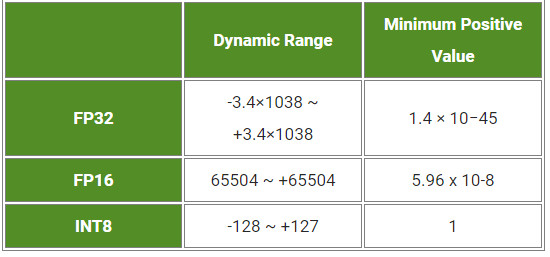trt_graph=trt.calib_graph_to_infer_graph(calibGraph)

在 NVIDIA Volta GPU 自動使用 Tensor 核心

NVIDIA Volta GPU Tensor 核心透過 TensorRT 進行半精準度 TensorFlow 模型推理，能提供相較於單精準度模型 8 倍的吞吐量。相較於更高精準度的 FP32 或 FP64，半精準度資料（FP16）能減少神經網路的顯存使用量，這使開發者能訓練和部署更大規模的神經網路，同時 FP16 相比 FP32 FP64，傳送時間更少。

Google 已發表 TensorFlow 1.7，同時也將跟 NVIDIA 更緊密地合作。希望這個新的解決方案能在提供最強效能的同時，保持 TensorFlow 的易用性和彈性。隨著 TensorRT 支援越來越多網路架構，大家只要更新就可以享受這些好處，無須改寫程式碼。

pip install tensorflow-gpu r1.7

（本文由 雷鋒網 授權轉載；首圖來源：TensorFlow